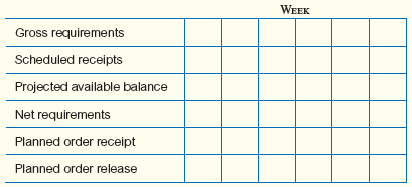# [Solved] Note: for These Problems, to Simplify Data Handling to Include

## Note: For these problems, to simplify data handling to include the receipt of orders that have actually been placed in previous periods, the following six-level scheme can be used. (A number of different techniques are used in practice, but the important issue is to keep track of what is on hand, what is expected to arrive, what is needed, and what size orders should be placed.) One way to calculate the numbers is as follows:One unit of A is made of three units of B, one unit of C, and two units of D. B is composed of two units of E and one unit of D. C is made of one unit of B and two units of E. E is made of one unit of F.Items B, C, E, and F have one-week lead times; A and D have lead times of two weeks.Assume that lot-for-lot (L4L) lot sizing is used for Items A, B, and F; lots of size 50, 50, and 200 are used for Items C, D, and E, respectively. Items C, E, and F have onhand (beginning) inventories of 10, 50, and 150, respectively; all other items have zero beginning inventory. We are scheduled to receive 10 units of A in Week 2, 50 units of E in Week 1, and also 50 units of F in Week 1. There are no other scheduled receipts. If 30 units of A are required in Week 8, use the low-level-coded bill-of-materials to find the necessary planned order releases for all components.

Tags

10+ million students use Quizplus to study and prepare for their homework, quizzes and exams through 20m+ questions in 300k quizzes.

Explore our library and get Decision Science Homework Help with various study sets and a huge amount of quizzes and questions

### Related Questions

Get Free Access Now!